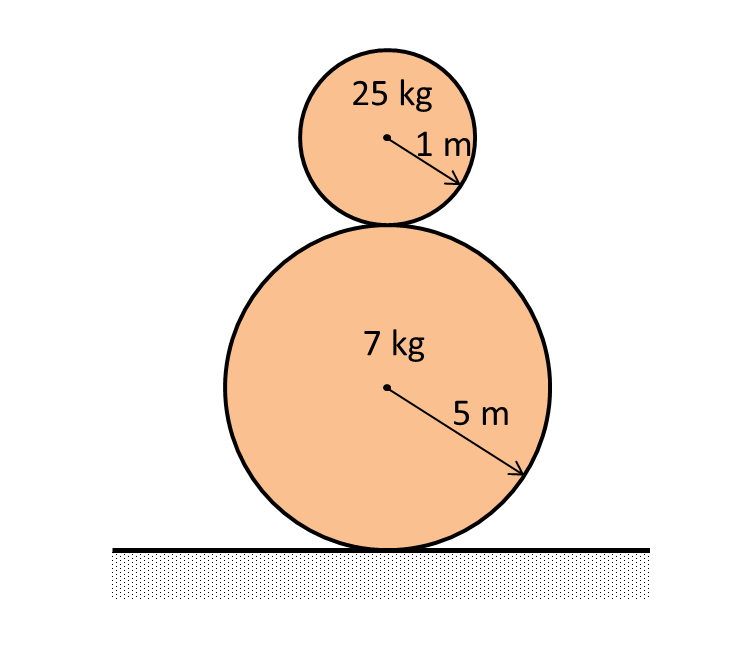# Challenges in mechanics by Ronak Agarwal (Part 1)A sphere of radius $1m$ and mass $25 Kg$ is put on another sphere of radius $5 m$ and $7 Kg$ which is placed on a smooth ground. Now the upper sphere is pushed very slightly from it's equilibrium position and it begins to fall.

Now when the line joining the centre of the 2 spheres makes an angle $\theta$ with the vertical the upper sphere loses contact with lower sphere. Then find $\theta$ in degrees to 1 decimal place.

Details and assumptions:

1)There is no friciton between the two spheres.

2)Take $g={ 9.8\quad m/s }^{ 2 }.$

×